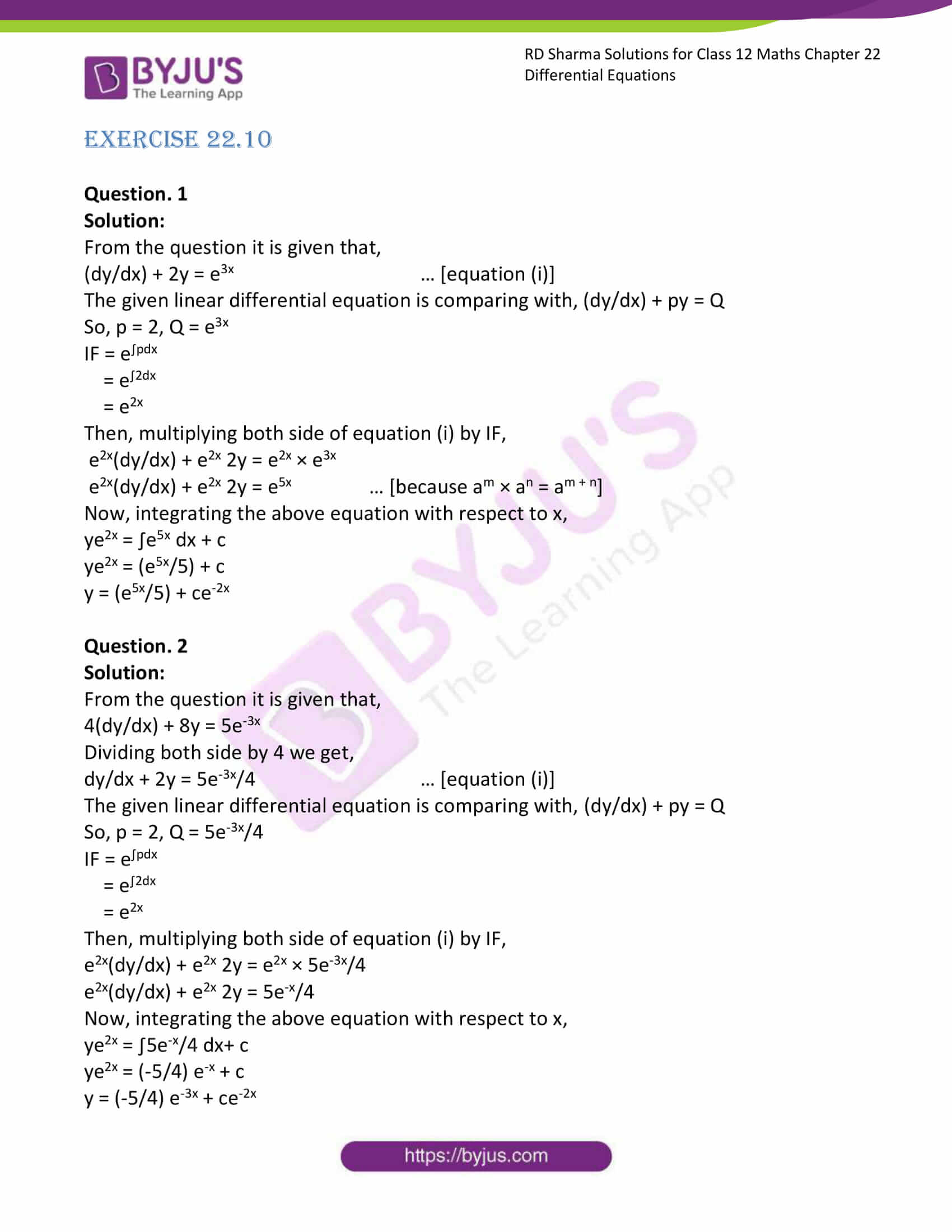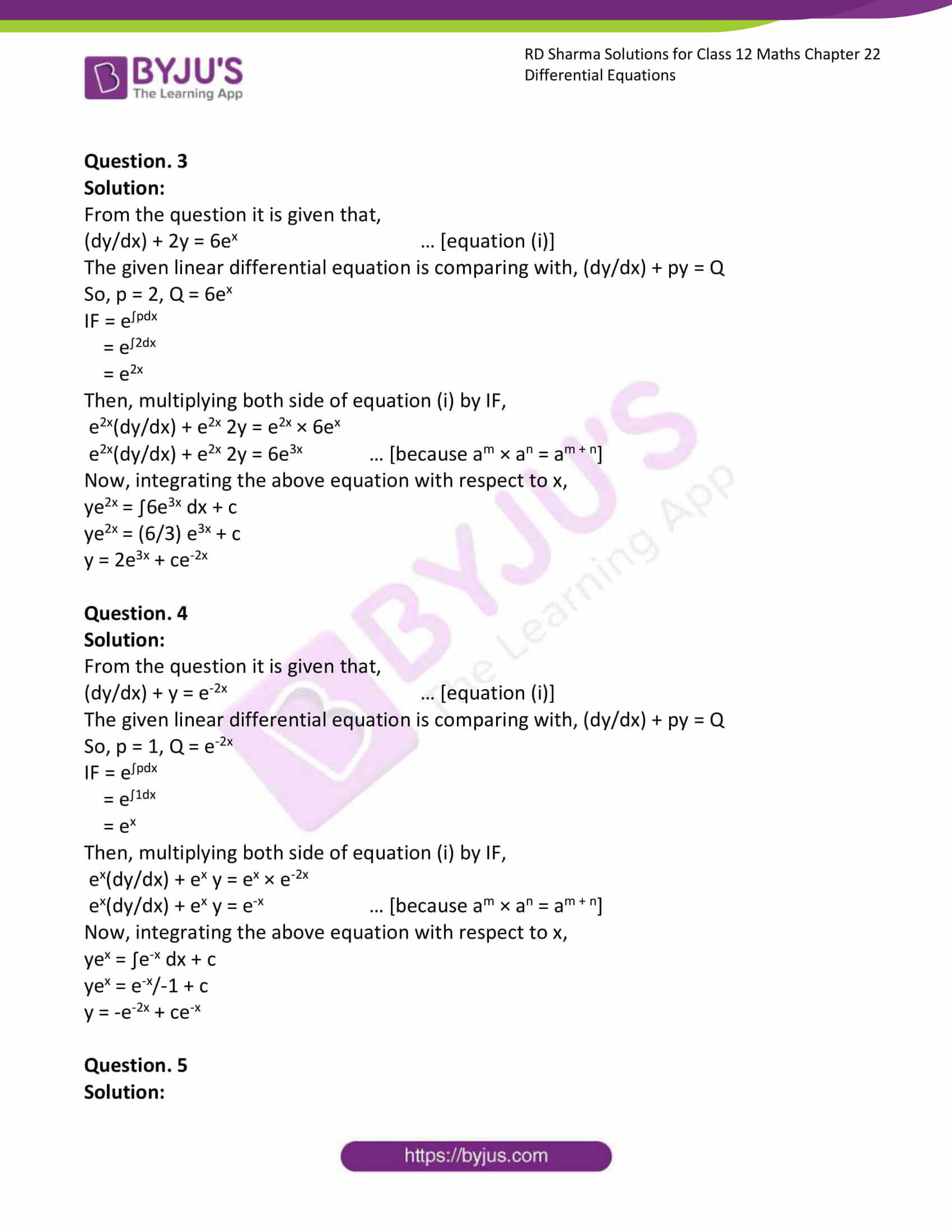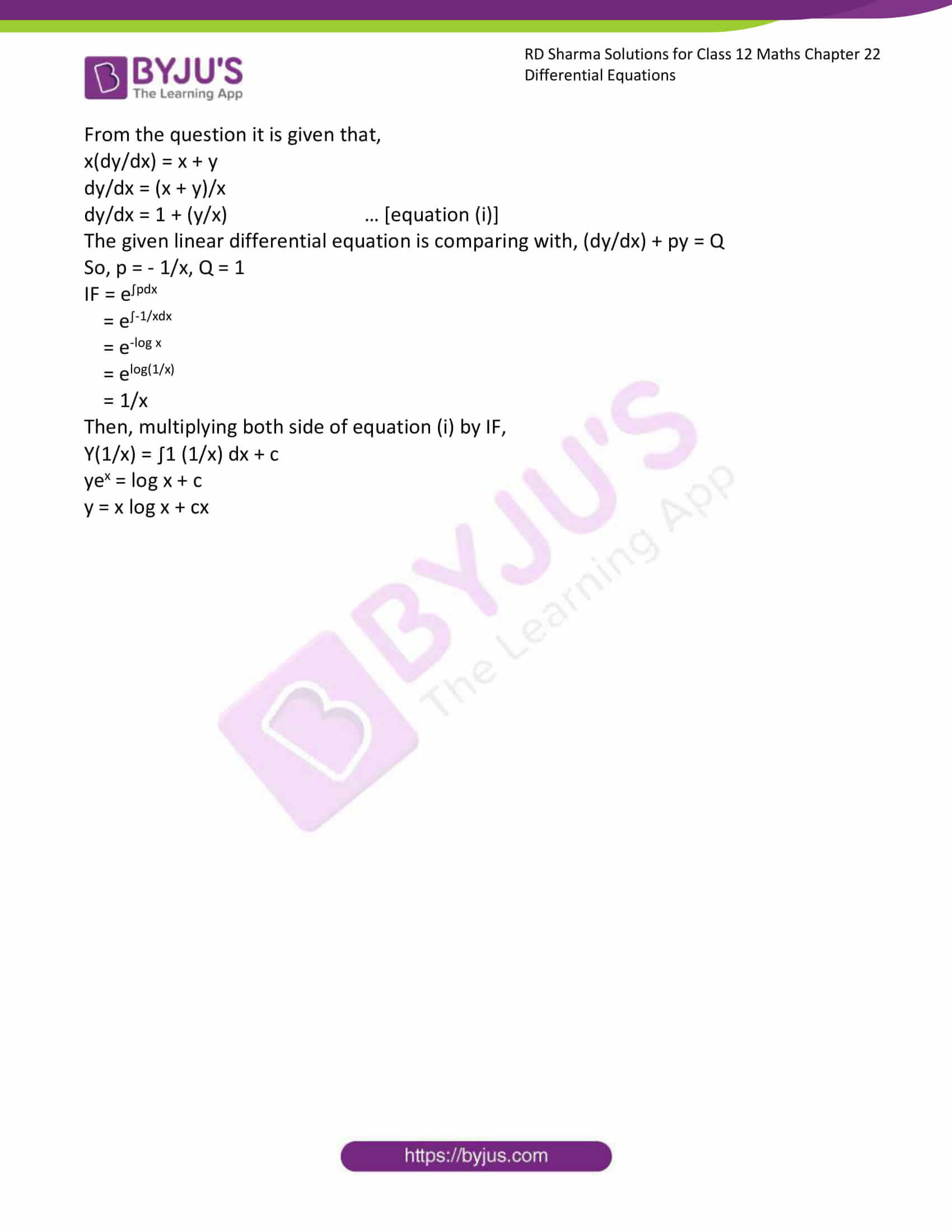# RD Sharma Solutions Class 12 Differential Equations Exercise 22.10

RD Sharma Solutions for Class 12 Maths Exercise 22.10 Chapter 22 Differential Equations are provided here. Our specialists will guide you in many methods to tackle questions on the exam and manage time in the examination. Students can refer to RD Sharma Solutions for Class 12 Maths Chapter 22 and practise various questions daily to perform well in the final exams.

## Download PDF of RD Sharma Solutions for Class 12 Maths Chapter 22 Exercise 10### Access RD Sharma Solutions for Class 12 Maths Chapter 22 Exercise 10

EXERCISE 22.10

Question. 1

Solution:

From the question it is given that,

(dy/dx) + 2y = e3x … [equation (i)]

The given linear differential equation is comparing with, (dy/dx) + py = Q

So, p = 2, Q = e3x

IF = e∫pdx

= e∫2dx

= e2x

Then, multiplying both side of equation (i) by IF,

e2x(dy/dx) + e2x 2y = e2x × e3x

e2x(dy/dx) + e2x 2y = e5x … [because am × an = am + n]

Now, integrating the above equation with respect to x,

ye2x = ∫e5x dx + c

ye2x = (e5x/5) + c

y = (e5x/5) + ce-2x

Question. 2

Solution:

From the question it is given that,

4(dy/dx) + 8y = 5e-3x

Dividing both side by 4 we get,

dy/dx + 2y = 5e-3x/4 … [equation (i)]

The given linear differential equation is comparing with, (dy/dx) + py = Q

So, p = 2, Q = 5e-3x/4

IF = e∫pdx

= e∫2dx

= e2x

Then, multiplying both side of equation (i) by IF,

e2x(dy/dx) + e2x 2y = e2x × 5e-3x/4

e2x(dy/dx) + e2x 2y = 5e-x/4

Now, integrating the above equation with respect to x,

ye2x = ∫5e-x/4 dx+ c

ye2x = (-5/4) e-x + c

y = (-5/4) e-3x + ce-2x

Question. 3

Solution:

From the question it is given that,

(dy/dx) + 2y = 6ex … [equation (i)]

The given linear differential equation is comparing with, (dy/dx) + py = Q

So, p = 2, Q = 6ex

IF = e∫pdx

= e∫2dx

= e2x

Then, multiplying both side of equation (i) by IF,

e2x(dy/dx) + e2x 2y = e2x × 6ex

e2x(dy/dx) + e2x 2y = 6e3x … [because am × an = am + n]

Now, integrating the above equation with respect to x,

ye2x = ∫6e3x dx + c

ye2x = (6/3) e3x + c

y = 2e3x + ce-2x

Question. 4

Solution:

From the question it is given that,

(dy/dx) + y = e-2x … [equation (i)]

The given linear differential equation is comparing with, (dy/dx) + py = Q

So, p = 1, Q = e-2x

IF = e∫pdx

= e∫1dx

= ex

Then, multiplying both side of equation (i) by IF,

ex(dy/dx) + ex y = ex × e-2x

ex(dy/dx) + ex y = e-x … [because am × an = am + n]

Now, integrating the above equation with respect to x,

yex = ∫e-x dx + c

yex = e-x/-1 + c

y = -e-2x + ce-x

Question. 5

Solution:

From the question it is given that,

x(dy/dx) = x + y

dy/dx = (x + y)/x

dy/dx = 1 + (y/x) … [equation (i)]

The given linear differential equation is comparing with, (dy/dx) + py = Q

So, p = – 1/x, Q = 1

IF = e∫pdx

= e∫-1/xdx

= e-log x

= elog(1/x)

= 1/x

Then, multiplying both side of equation (i) by IF,

Y(1/x) = ∫1 (1/x) dx + c

yex = log x + c

y = x log x + cx